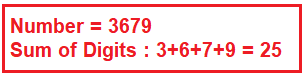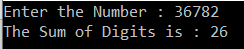# Sum of Digits Program in C#

## Sum of Digits Program in C# with Examples:

In this article, I am going to discuss the Sum of Digits Program in C# with Examples. Please read our previous article where we discussed the Factorial Program in C# in many different ways. This is one of the frequently asked interview questions. As part of this article, we will discuss the following things.

1. How to find the sum of digits of a given number using a loop?
2. How to find the sum of digits of a given number using recursion?
3. Using LINQ to find the sum of digits of a number in C#.
##### Finding the sum of digits of a given number using a loop in C#:Let us first understand the algorithm to find the sum of digits of a number.

##### Algorithm: Sum of Digits Program in C#

Step1: Get the number from the user
Step2: Get the modulus/remainder of that number by doing the modulus operation
Step3: Sum the remainder of the number
Step4: Divide the number by 10
Step5: Repeat step 2 while the number is greater than 0.

##### Let us put the above steps into a program.
```using System;
namespace LogicalPrograms
{
public class Program
{
static void Main(string[] args)
{
Console.Write("Enter the Number : ");
int sum = 0, reminder;

while (number > 0)
{
reminder = number % 10;
sum = sum + reminder;
number = number / 10;
}

Console.WriteLine(\$"The Sum of Digits is : {sum}");
}
}
}
```
###### Output:##### Finding the sum of digits of a number using Recursion in C#:

Let us understand how to find the sum of digits of a number using Recursion in C#. Please have a look at the following program.

```using System;
namespace LogicalPrograms
{
public class Program
{
static void Main(string[] args)
{
Console.Write("Enter the Number : ");
int sum = SumOfDigits(number);
Console.WriteLine(\$"The Sum of Digits is : {sum}");
}

static int SumOfDigits(int number)
{
if (number != 0)
{
return (number % 10 + SumOfDigits(number / 10));
}
else
{
return 0;
}
}
}
}
```
###### Output:##### Using Linq to find the sum of digits of a number in C#:

In the following program, first, we convert the integer into a string. As we know a string is an array of characters, so we use the Select operator to iterate over the array. For each character digit, first, we parse it to a string and then we parse it to an int. Send the resulting enum to an int[] array. And then apply the Sum extension method to it to get the desired result.

```using System;
using System.Linq;

namespace LogicalPrograms
{
public class Program
{
static void Main(string[] args)
{
Console.Write("Enter the Number : ");

int sum = number.ToString().Select(digit => int.Parse(digit.ToString())).ToArray().Sum();

Console.WriteLine(\$"The Sum of Digits is : {sum}");